# Precalculus : Find the Measure of a Coterminal Angle

## Example Questions

### Example Question #1 : Find The Measure Of A Coterminal Angle

Find the coterminal angle of 15 degrees.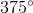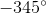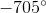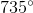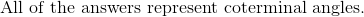Explanation:

The coterminal angles can be positive or negative.  To find the coterminal angles, simply add or subtract 360 degrees as many times as needed from the reference angle.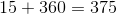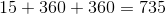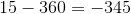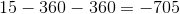All of these angles are coterminal angles.

### Example Question #3 : Angles In The Coordinate Plane

Of the given answers, what of the following is a coterminal angle ofradians?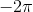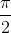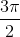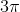Explanation:

To find the coterminal angle of an angle, simply add or subtractradians, or 360 degrees as many times as needed.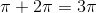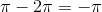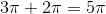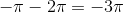These are all coterminal angles toradians.

Out of the given answers,is the only possible answer.

### Example Question #1 : Find The Measure Of A Coterminal Angle

Of the following choices, find a coterminal angle of.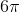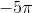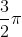Explanation:

In order to find a coterminal angle, simply add or subtractradians to the given angle as many times as possible.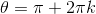The possible angles after adding increments ofradians are: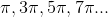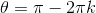The possible angles after subtracting decrements ofradians are: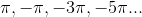Out of the given possibilities, onlyis a valid answer.

### Example Question #4 : Find The Measure Of A Coterminal Angle

Find the coterminal angle of 15 degrees in standard position from the following answers.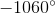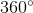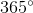Explanation:

To determine the coterminal angle, simply add or subtract increments or decrements of 360 degrees to the given angle.

For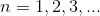: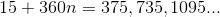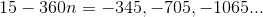These angles can all be coterminal to 15 degrees.  The only answer is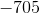.

### Example Question #1 : Find The Measure Of A Coterminal Angle

Find the coterminal angle of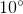, if possible.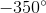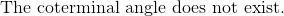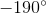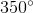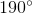Explanation:

In order to find a coterminal angle, or angles of the given angle, simply add or subtract 360 degrees of the terminal angle as many times as possible.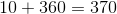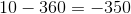The only correct answer is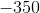.

### Example Question #1 : Find The Measure Of A Coterminal Angle

Which of the following angles is coterminal to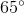?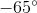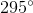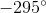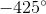Explanation:

Which of the following angles is coterminal to?

Coterminal angles are angles which start and end at the same point. In other words, they share both their starting and ending point. Note, this doesn't require them to be the same angle.

For instance,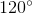is coterminal with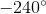, because they both start on the positive x-axis, and end at the same place in quadrant 2.

So, we want to find an angle that ends at the same place in quadrant 1 as. Of the answer choices, only 1 ends in quadrant 1, so that one must be our answer:### All Precalculus Resources